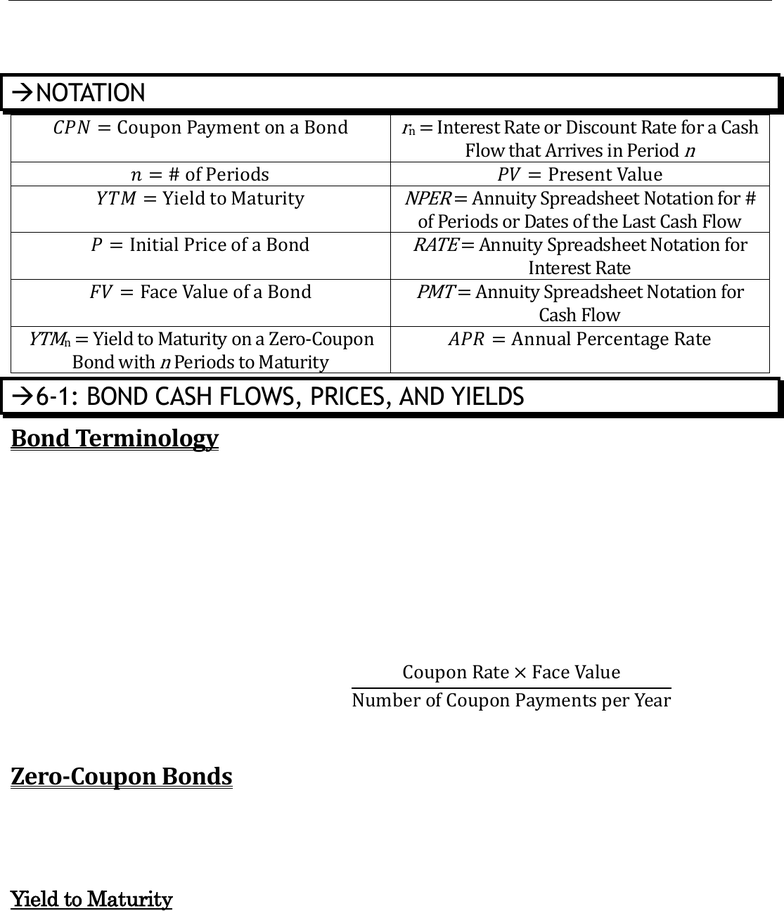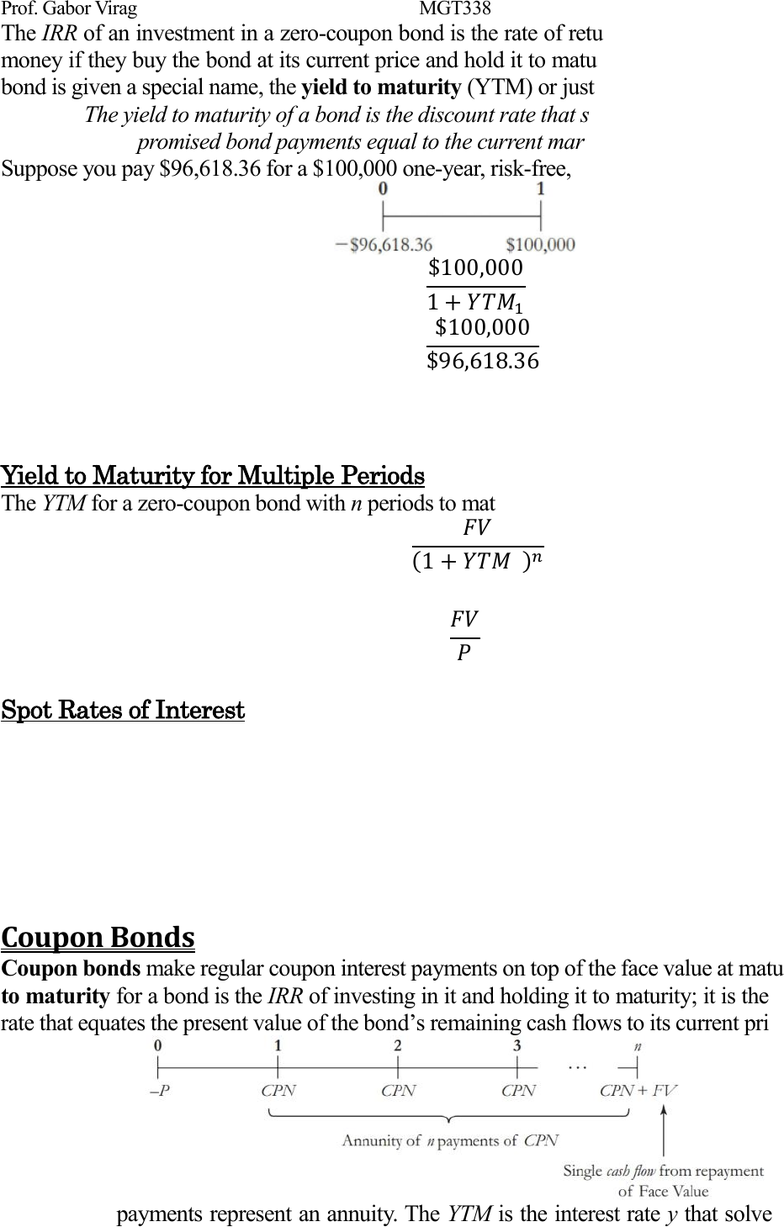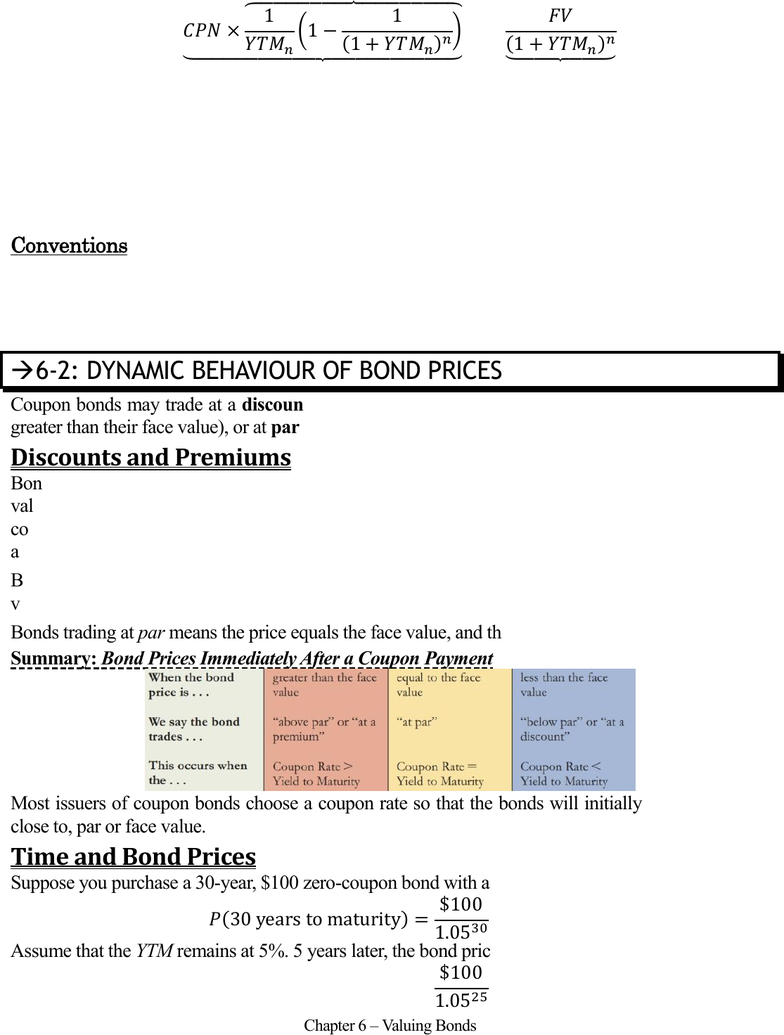Textbook Notes (280,000)
CA (170,000)
UTM (8,000)
Chapter 6

# MGT338H5 Chapter Notes - Chapter 6: Notional Amount, Government Bond, Yield CurveExam

Department
Management
Course Code
MGT338H5
Professor
Gabor Virag
Chapter
6

This preview shows pages 1-3. to view the full 9 pages of the document.Prof. Gabor Virag MGT338 Page 1 of 9
Chapter 6 Valuing Bonds
Chapter 6 Valuing Bonds
Bond Cash Flows, Prices, and Yields; Dynamic Behaviour of Bond Prices;
Yield Curve and Bond Arbitrage; Corporate Bonds; and Sovereign Bonds
NOTATION
 

 


 
 
6-1: BOND CASH FLOWS, PRICES, AND YIELDS
Bond Terminology
A bond is a security sold by governments & corporations to raise money from investors in exchange for
promised future payment. The terms of the bond are described as part of the bond indenture, which
indicates the amounts & dates of all payments to be made. These payments are made until a final
repayment date, the maturity date. The time left till repayment is the term of a bond.
Bonds typically make 2 types of payments to their holders. The promised interest payments of a bond
are called coupons. The principal or face value of a bond is the notional amount we use to compute
the coupon payments. Usually, the face value is repaid at maturity. The amount of each coupon payment
is determined by the coupon rate, which is the percentage of the face value that is paid as coupons
each year.


Example: A \$1000 bond with a 10% coupon rate and semiannual paymentswill pay coupon
payments of (10% × \$1000)/2 = \$50 every six months.
Zero-Coupon Bonds
The simplest type of bond is a zero-coupon bond, a bond that does not make coupon payments and
only pays the face value at maturity. Treasury Bills, which are Government of Canada bonds with a
maturity of up to one year, are zero-coupon bonds. Zero-coupon bonds always trade at a discount (a
price lower than the face value), so they are also called pure discount bonds.
Yield to Maturity
The IRR of an investment opportunity is the discount rate at which the NPV of the investment
opportunity is equal to zero.

Only pages 1-3 are available for preview. Some parts have been intentionally blurred.Prof. Gabor Virag MGT338 Page 2 of 9
Chapter 6 Valuing Bonds
The IRR of an investment in a zero-coupon bond is the rate of return that investors will earn on their
money if they buy the bond at its current price and hold it to maturity. The IRR of an investment in a
bond is given a special name, the yield to maturity (YTM) or just the yield:
The yield to maturity of a bond is the discount rate that sets the present value of the
promised bond payments equal to the current market price of the bond.
Suppose you pay \$96,618.36 for a \$100,000 one-year, risk-free, zero-coupon bond.
 


  
   
Thus, by the Law of One Price, the competitive market risk-free interest rate is 3.5%. That means all
one-year, risk-free investments must earn 3.5%.
Yield to Maturity for Multiple Periods
The YTM for a zero-coupon bond with n periods to maturity, current price P, and face value FV is:
  

Rearranging, we get the Yield to Maturity of an n-Year Zero-Coupon Bond:


This is the effective rate of return per period for holding the bond from today until maturity on date n.
Spot Rates of Interest
The spot rate of interest is the risk-free interest rate. Because a default-free zero-coupon bond that
matures on date n provides a risk-free return over the same period, the Law of One Price guarantees
that the spot rate of interest equals the yield to maturity on such a bond.
Risk-Free Interest Rate (Spot Rate of Interest) with Maturity n

The yield curve plots the risk-free interest rate for different maturities, which corresponds to the yields
of risk-free zero-coupon bonds. Thus, the yield curve is also called the zero-coupon yield curve.
Coupon Bonds
Coupon bonds make regular coupon interest payments on top of the face value at maturity. The yield
to maturity for a bond is the IRR of investing in it and holding it to maturity; it is the single discount
rate that equates the present value of the bonds remaining cash flows to its current price.
The coupon payments represent an annuity. The YTM is the interest rate y that solves the following
equation for the Yield to Maturity of a Coupon Bond.

Only pages 1-3 are available for preview. Some parts have been intentionally blurred.Prof. Gabor Virag MGT338 Page 3 of 9
Chapter 6 Valuing Bonds
  










Unfortunately, there is no simple formula to solve for the YTM directly. We need to use a financial
calculator, trial and error, or the annuity spreadsheet. When the equation is solved, the yield will be an
effective rate per coupon interval. This yield is typically multiplied by the number of coupons per year
and stated as an annual rate as an APR.
If we know the YTMn, we can calculate the price of the bond using the formula above.
Conventions
We can convert any price into a yield, so yield & prices are interchangeable. Bonds are often quoted
with their YTM, since it easier to use for comparisons. Prices can be quoted as well, such as \$944.98
per \$1000 face value. To make it easier to compare, prices are often quoted as a percentage of their
face value; 94.498.
6-2: DYNAMIC BEHAVIOUR OF BOND PRICES
Coupon bonds may trade at a discount (a price lower than their face value), at a premium (a price
greater than their face value), or at par (a price equal to their face value).
Bonds trading at a discount mean an investor will earn a return from both the coupons and the face
value. Its YTM exceeds its coupon rate. The reverse is also true; If a coupon bond’s YTM exceeds its
coupon rate, the PV of its cash flows at the YTM will be less than its face value, and the bond will trade
at a discount.
Bonds trading at a premium mean an investors return from coupons is diminished by receiving a face
value less than the price paid for the bond. It’s YTM is less than its coupon rate.
Bonds trading at par means the price equals the face value, and the YTM is equal to the coupon rate.
Summary: Bond Prices Immediately After a Coupon Payment
Most issuers of coupon bonds choose a coupon rate so that the bonds will initially trade at, or very
close to, par or face value.
Time and Bond Prices
Suppose you purchase a 30-year, \$100 zero-coupon bond with a yield to maturity of 5%. Initially

  
Assume that the YTM remains at 5%. 5 years later, the bond price will be

  